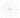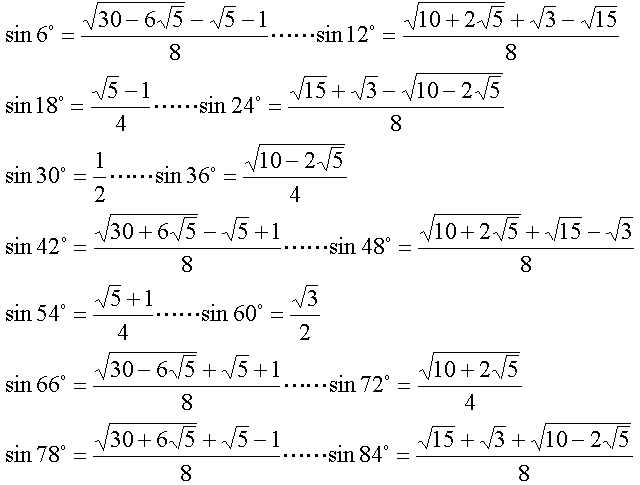## 无理角度

Sat, 14th December 2019Edit on Github无理数角度

# 摘要

mathe于2008年5月提问

zeroieme于2011年4月 提问:

$\frac{\theta}{\pi}$$\cos(\theta)$都是有理数，证明$\cos(\theta)$必为下列几个数之一：
$-1,-\frac12,0,\frac12,1$

hejoseph于2016年12月 提问:
$a$ 是有理数，$0\leq a\leq 90$
$\cos a^{\circ}$ 也是有理数，则 $a=0$$a=60$$a=90$
$\cos a^{\circ}$ 是一个不可约整系数二次方程的根，则 $a=30$$a=36$$a=45$$a=72$
$\cos a^{\circ}$ 是一个不可约整系数三次方程的根，则 $a$ 可能是哪些值呢？

# 详细内容

zgg__认为，是无理角度吧。因为sin值是$\sqrt{\frac{\sqrt{3}}2}$，那么$(\cos(\theta)+i\sin(\theta))^n$的虚部有4次$\sqrt{3}$捣乱，总是没法等于0。所以，$(\cos(\theta)+i\sin(\theta))^n$不能为1，角度也就是无理角了吧。
mathe建议采用第二类切皮雪夫多项式 来解决:

$U_n(x)=\left|\begin{matrix}2x&1&0&0&\dots&0&0\\ 1&2x&1&0&\dots&0&0\\ 0&1&2x&1&\dots&0&0\\ 0&0&1&2x&\dots&0&0\\ \cdots&\cdots&\cdots&\cdots&\ddots&\cdots&\cdots\\ 0&0&0&0&\cdots&2x&1\\ 0&0&0&0&\cdots&1&2x\end{matrix}\right| =2^n\prod_{k=1}^n (x-\cos(\frac{k\pi}{n+1}))$

$U_n(\frac{\sqrt{3}-1}2)=\left|\begin{matrix}\sqrt{3}-1&1&0&0&\dots&0&0\\ 1&\sqrt{3}-1&1&0&\dots&0&0\\ 0&1&\sqrt{3}-1&1&\dots&0&0\\ 0&0&1&\sqrt{3}-1&\dots&0&0\\ \cdots&\cdots&\cdots&\cdots&\ddots&\cdots&\cdots\\ 0&0&0&0&\cdots&\sqrt{3}-1&1\\ 0&0&0&0&\cdots&1&\sqrt{3}-1\end{matrix}\right|\ne 0$

zgg__继续细化其思路 ，认为:

$z=\cos(\theta)+i\sin(\theta)$的一个零化多项式是$f(z)=(z^2+z+1)^2-3z^2$,

liangbch贴出了6°角倍数的正弦值:mathe说明有了上面这些角已经足够了，因为

$T_n(x)$是第一类切皮雪夫多项式,

$S_0(x)=2,S_1(x)=x, S_{n+1}(x)=xS_n(x)-S_{n-1}(x)$.

$S_{2v}(2\cos(q\pi))=2\cos(2u\pi)=2$.

Github